Page 3 model: Woollen jumpers

As the weather turns from grey to cold and grey, it’s time to get out your favourite woolly jumper. Only, it’s a bit longer than you remember. Has someone has been stretching it out behind your back? Instead of jumping to conclusions, let’s try to understand how this wool-d happen.Your jumper is made up of yarn, made from woollen fibres spun together. Let’s assume the fibres are in one of two states: $A$, unstretched, or $B$, completely stretched out.
The jumper as a whole is much harder to stretch with the fibres in state $B$ than in state $A$, because each individual fibre is already elongated.

As we start stretching the jumper, more and more fibres go from state $A$ to state $B$, and the rate at which it stretches slows down. Let’s model this stretching over time as
$$\frac{\mathrm{d}\ell}{\mathrm{d}t} = \alpha-\beta l,$$
where $t$ is time, $\ell$ is how much the jumper has stretched, and $\alpha$, $\beta$ are constants depending on the mechanical properties in states $A$ and $B$.Assume that the jumper started off un-stretched (unless you got fleeced), so that $l(0)=0$. Then this equation has a unique solution, giving an explicit formula for how much your jumper has stretched:
$$\ell(t) = \frac{\alpha}{\beta}\left( 1- \frac{1}{\exp \left( \beta t \right)} \right).$$
On the bright side, even if someone has been wearing it, this shows your jumper won’t go on stretching forever: as $t$ gets very large, $\ell \approx \alpha/\beta$.

This approximate solution was reproduced by experimental evidence from the appropriately named Wool Textile Research Laboratory, just in case you thought this whole ‘two-state’ yarn was a bit of a stretch.Page 3 model: Cows

Have you ‘herd’? The world’s largest cow is over six feet tall and weighs more than 1.3 tonnes. Is a bigger cow possi-bull? Will the future contain infinitely large cows? The steaks have never been higher!

To answer this question, let’s take a look at the cow’s legs. If the main (meaty) bit of the cow has a volume $V$ and density $\rho$ then its weight is $\rho V g$. So each leg supports a load of about
$N = \frac{\rho V g}{4}.$
In pursuit of glory, let’s now make the length, height and width of the cow bigger by a factor $a$. The cow’s new volume is $a^3 V$ and so the load on each leg is $a^3N$: it grows cubically as $a$ increases.

Can the legs cope? If we model the legs as cylinders (since they already ‘lactose’…), we can use a 1757 result from the famous cow enthusiast Euler: if a cylinder has height $L$ and radius $r$, the maximum load it can support standing upright is
$N_\text{max} = \frac{E \pi^3 r^4}{4 L^2}.$
$E$ here is just a property of the material: its stiffness, or Young’s moo-dulus.With our scaling, $L$ and $r$ are now $a$ times bigger. Our new maximum load is
$\frac{E\pi^3a^4r^4}{4a^2 L^2} = a^2 N_\text{max}.$

Uh oh… this only scales as $a^2$: quadratically.

So even though $N_\text{max}$ starts above $N$ (it has to, given that these cows exist!), there will come a maximum possible $a$, after which there will beef-ar too much cow and its legs will give way… an udder disaster.

This analysis tells us something really important about biology—that there is a natural maximum size for land mammals. But have we reached it for cows? Brody & Lardy’s 1000-page tome Bioenergetics and Growth from 1946 has all the de-tail you need. We’ll leave you to ruminate on the cow-culations.Page 3 model: Solitons

Solitons are special analytic solutions to the nonlinear wave equations that turn up everywhere: from fluid dynamics to quantum mechanics to molecular biology. One such equation is the Korteweg–de Vries (KdV) equation for a 1D wave $u(x,t)$ evolving in time $t$: $\frac{\partial u}{\partial t}+6u\frac{\partial u}{\partial x}+\frac{\partial^3 u}{\partial x^3}=0.$ What makes solitons special is that they behave in many ways like solutions to the linear wave equation: propagating without losing their shape, and even interacting nicely:In 1990, Daisuke Takahashi and Junkichi Satsuma proposed a discrete model for solitons called the ball and box model. It consists of an infinite row of boxes, some of which contain a ball. A sequence of consecutive balls represents a wave. The pattern of waves after each time step is found by starting from the left and moving each ball to the next available empty box to its right.A row of $n$ consecutive balls behaves like a single wave, and moves to the right at a constant speed $n$. Several such waves, so long as they start sufficiently separated, will interact (in a possibly messy way), and then disperse, maintaining their original shapes overall.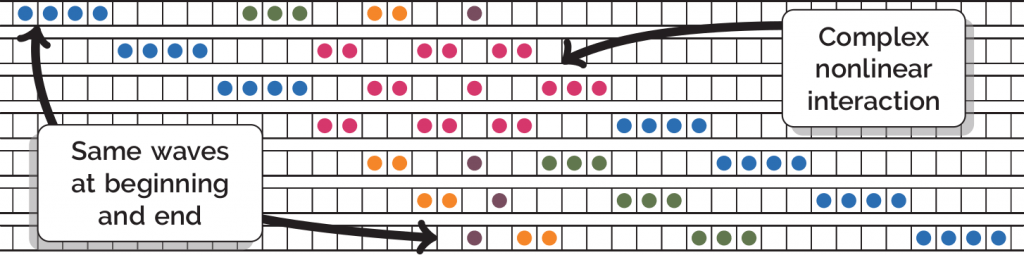This model is fun to play with, but—surprisingly given its simplicity—it manages to capture a large amount of the interesting and unusual behaviour of the fluid waves modelled by the KdV equation.Page 3 model: Cooking spaghetti

You there! Yes—you cooking the spaghetti! How do you tell when it’s cooked? No, don’t throw it against the wall… maths is here for you.

Cooking pasta increases the amount of water it contains until the pasta is fully hydrated, at which point we say it’s cooked. But this means that the pasta is no longer a rigid material—it’s flexible.

Nathaniel Goldberg and Oliver O’Reilly, in their 2020 paper, model the process of spaghetti cooking in a saucepan:Measure the arclength, $s$, of your spaghetto from $s=0$ to $s=L$ and call the angle to the horizontal at any point $\theta(s)$:Flexible rod theory tells you that the moment along the spaghetto, $\boldsymbol{m}$, depends on the angle, $\theta$, and the weight force, $\boldsymbol{n}$. For a bent spaghetto, you can write the curvature, $\kappa$, in terms of the intrinsic curvature, $\kappa_0$, and the moment, divided by the rigidity, $EI$: $\frac{\partial\theta}{\partial s} = \kappa = \kappa_0 + \frac{m}{EI}.$

In the pan!

But the intrinsic curvature changes as water is absorbed. Goldberg & O’Reilly use a model from plant stem growth for the rate of intrinsic curvature change, making it dependent on the amount of time the spaghetto has been in the water for:

$\frac{\partial \kappa_0}{\partial t} = \alpha(t)(\kappa – \kappa_0) \quad \text{where} \quad \alpha(t) = \alpha_{\infty}\frac{1-\mathrm{e}^{-t/\tau}}{1+\mathrm{e}^{-(t-t_0)/\tau}}.$

Want the spaghetti al dente? Better get working out the parameters $\alpha_\infty$ and $\tau$…

References

1. NN Goldbery & OM O’Reilly Mechanics-based model for the cooking-induced deformation of spaghetti, Physical Review E, 101, 03001, (2020).Page 3 model: Bees

If you model rabbits under ideal circumstances, you may find that the number of pairs of rabbits each month follows the Fibonacci sequence.

In this case, ‘ideal circumstances’ is a euphemism for nonsense, as your assumptions would include blatant untruths such as “rabbits mate once a month every month except their first month alive”, “a pair of rabbits gives birth to exactly one pair of rabbits per month”, and “the hutch is infinitely big (and hence Starsky is very squashed)”.

Fibonacci numbers, however, are not completely absent from nature. They accurately describe a vastly superior animal: the honeybee.

Male bees (drones) come from unfertilised eggs, and so they only have one parent — the queen.
Female bees (workers or queens) come from fertilised eggs and so have two parents — the current queen and a drone.

If you follow a drone’s family tree backwards, you will see that a drone has:The number of ancestors of a male bee follows the Fibonacci sequence.

Who would’ve expected that?!Page 3 model: Game of Thrones

One of the best parts of getting into a series is getting to know and love the main characters. However, in Game of Thrones (or A Song of Ice and Fire, for you purists), this can be a heart-breaking activity. Who will survive to the end and who will bite the dust? No one knows, but perhaps maths can lend a hand.Image: Andrew Beveridge

Andrew Beveridge and Jie Shan used network theory to investigate who the main characters of Game of Thrones are. The diagram above shows all the interactions between characters during the seventh series: the larger characters are more central, as determined by the PageRank algorithm.

However, it only takes one swing of an axe to drastically change the network…Page 3 model: Ponytails

The ponytail hairstyle is a synonym of comfort and simplicity, and what was once considered a traditional schoolgirl style, nowadays it has become popular again thanks to clever styling. But trying to work out what shape someone’s ponytail will be has puzzled scientists and artists since Leonardo da Vinci.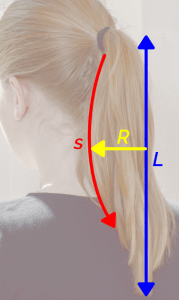In 2012, scientists from the University of Cambridge and University of Warwick developed the ponytail shape equation (PSE) to unravel some of the mysteries of the ponytail. Their model takes into account the gravity ($g$), the elasticity of the hair, the presence of random curliness of hair, and an outward swelling pressure that arises from collisions between the component hairs (which explains how a bundle of hair is swelled).

This equation can be used to find $R$, the radius of the ponytail, in terms of $s$, the arc length along the ponytail. The length at which gravity bends the hair is $l$, $L$ is the length of the ponytail, $P$ is the pressure due to the hairband, $A$ is the bending modulus, and $\rho$ is the hair’s density.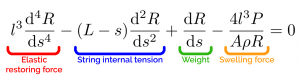The Rapunzel number, $\text{Ra}$, of a ponytail is the ratio $L/l$. This dimensionless number determines the effect of gravity on hair. When $\text{Ra}<1$, the hair doesn’t bend much, leading to a thin, straight ponytail. When $\text{Ra}>1$, the hair bends strongly under gravity leading to a wide, bushy ponytail.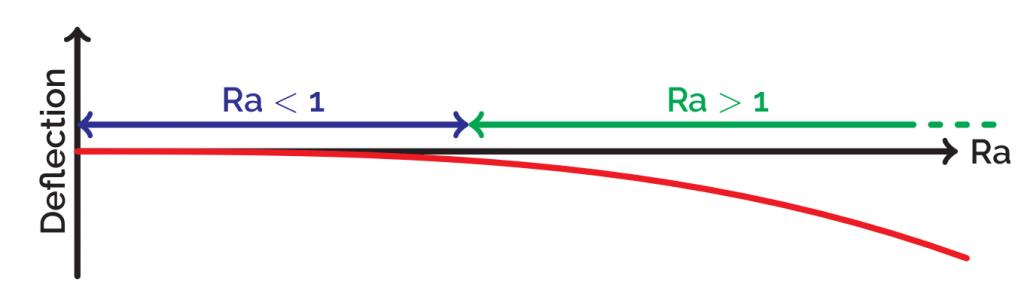The relevance of this equation is that it could help in understanding the structure of materials made up of fiber and depicting hair realistically in animation and video games. But most importantly, if you want to look good at a party or a maths conference, simply calculate your Rapunzel number and pop on a hairband that exerts the correct pressure.

References

1. RE Goldstein, PB Warren, RC Ball, Shape of a Ponytail and the Statistical Physics of Hair Fiber Bundles, Physical Review Letters, 108, 078101, (2012).
2. RE Goldstein, (2016, September 11), Leonardo, Rapunzel and the Mathematics of Hair.Page 3 model: Frictional unemployment

If I had a pound for every time someone assumed I studied maths because I wanted to be an economist without writing essays, I’d have enough to make it worth following the stock market. However, once the indignation fades, I can see the attraction—there are a lot of interesting uses of mathematics in economics. One of the most basic, yet most important, is modelling unemployment.

Unemployment might be caused by too few jobs in an area. Or, it may also be due to a lack of information being provided to employers or potential workers: there may be perfectly good jobs available that qualified workers simply don’t know about. This sort of unemployment is called frictional unemployment.

We split the labour force $L$ into two separate populations: employed ($N$) and unemployed ($U$). We then define $s$ and $f$ to be the rates at which people gain and lose employment:The rate of change in unemployment is:
\begin{align*}
\frac{\text{d} U}{\text{d}t}&=\text{number becoming unemployed} -\text{number entering work}\\
&=sN(t)-fU(t)
\end{align*}
If we assume that the total size of the labour force is constant, then this leads us to:
$$\frac{\text{d}u}{\text{d}t}+(s+f)u=s,$$
where $u$ is the proportion of the labour force that is unemployed. A lovely first order ODE, which can be solved using the integrating factor method (an exercise left for the reader). Simple enough that even an economist would understand!Page 3 model: Crowd control

Being part of a crowd is something that we all have to experience from time to time. Whether it’s in a busy shop or commuting to work, the feeling of being swept along by those around us is all too familiar. The ubiquity of the situation, and the huge amount of data available from CCTV footage, makes crowd dynamics a favourite subject for mathematical modelling.

One popular method is known as the social force model, which applies Newton’s second law to each member of the crowd. Each individual accelerates to maintain their ‘desired velocity’, and this is balanced against forces from physical obstacles as well as the social force that maintains polite distance between people—a mathematical interpretation of personal space!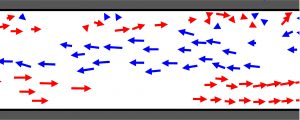Lanes naturally form when people walk in opposite directions. Image: Dirk Helbing and Peter Molnar

Huge simulations of up to a million pedestrians have been run, which show the model’s remarkable powers. If groups of people want to travel in opposite directions along a bridge, for example, lanes of alternating direction naturally form to minimise “bumping”.When two crowds meet at a gap, the walking direction oscillates. Image: Dirk Helbing and Peter Molnar

Some of the results are more unexpected. For example, if people try and move too fast then it can actually slow them down via an increase in ‘friction’ that results from pushing. Further, it can be shown that two narrow doors are a more effective way of leaving a room than one big door, so putting a bollard in the middle of an exit actually speeds people up!

Still, not much solace when you’re stuck in a Christmas scramble at Woolworths…

References

Helbing D and Molnar P (1997). Self-organization phenomena in pedestrian crowds. In: Schweitzer F (ed.) From individual to collective dynamics, 569–577.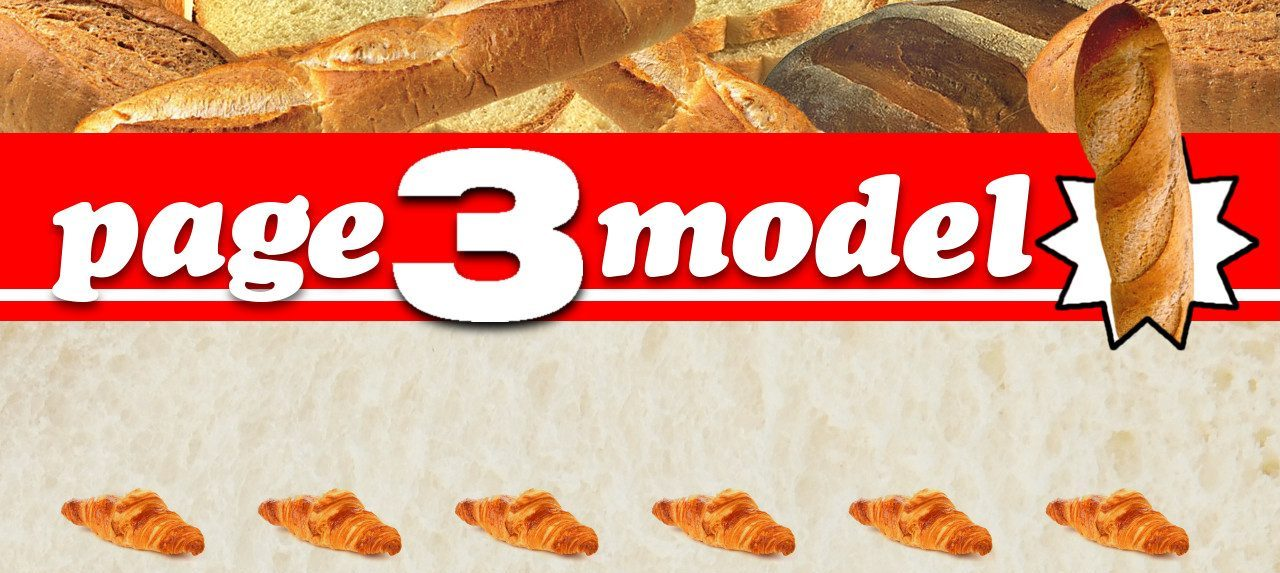Page 3 model

Bread is a staple of many diets. From delicious garlic bread to crunchy pizza, it’s enjoyed throughout the world. But have you ever wondered what mathematics lies just beneath the crust?  Thankfully DR Jefferson, AA Lacey and PA Sadd at Heriot-Watt University have! No? Well, we’re going to tell you anyway.

Bread dough is initially a bubbly liquid, with bubbles connected to other bubbles in a ‘matrix’.  These bubbles will collapse, provided that both the temperature and temperature gradient are high enough. To start with, the bubbles at the surface (which is hotter than the interior) reach a temperature at which they are likely to fracture. At this point, the temperature gradient is also high, with plenty of cooler liquid dough nearby. However, when the temperature of the interior has increased sufficiently to allow the bubbles inside to burst, the temperature gradient is much lower, the matrix has set, there is less liquid dough nearby, and so less collapse can take place.

But that’s not all! We can refine the model by considering the movement of the ‘crust boundary’ (where bubbles collapse) as the dough rises, as well as the vaporisation of moisture inside the bubbles. Both of these allow for the transfer of heat and affect the thermodynamics of the whole process.So in the future, please try to remember all the maths that worked hard to ensure the crustiness of your bread! And, on that note, we’re off to get pizza…

References

Jefferson DR, Lacey AA & Sadd PA 2007 Crust density in bread baking: Mathematical modelling and numerical solutions. Applied Mathematical Modelling 31 (2) 209–225.
Jefferson DR, Lacey AA & Sadd PA 2007 Understanding crust formation during baking. Journal of Food Engineering 75 (4) 515–521.